# EXAMPLE 1 Graph a quadratic inequality Graph y

• Slides: 8EXAMPLE 1 Graph a quadratic inequality Graph y > x 2 + 3 x – 4. SOLUTION STEP 1 Graph y = x 2 + 3 x – 4. Because the inequality symbol is >, make the parabola dashed. STEP 2 Test a point inside the parabola, such as (0, 0). y > x 2 + 3 x – 4 ? 0 > 02 + 3(0) – 4 0>– 4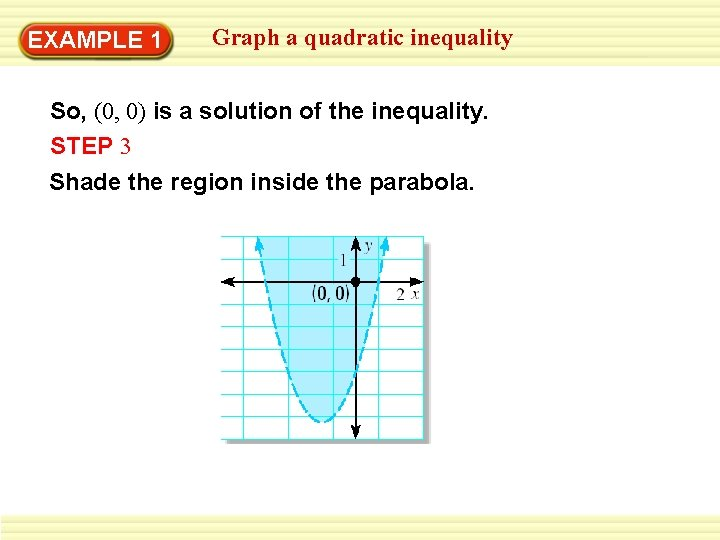EXAMPLE 1 Graph a quadratic inequality So, (0, 0) is a solution of the inequality. STEP 3 Shade the region inside the parabola.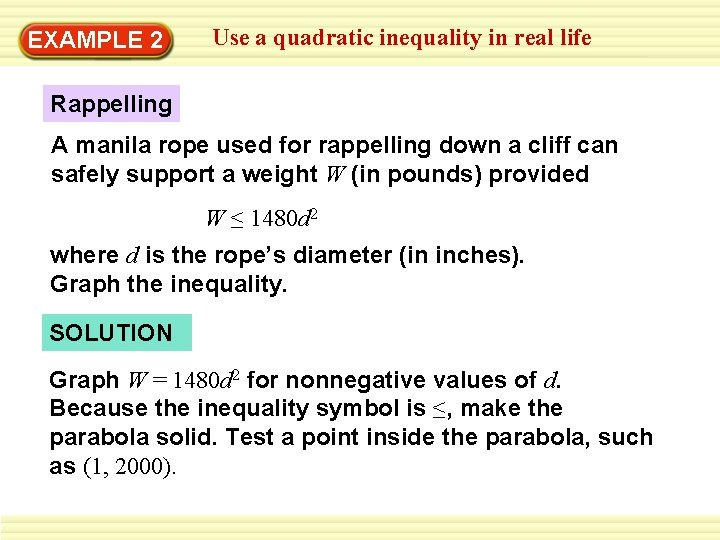EXAMPLE 2 Use a quadratic inequality in real life Rappelling A manila rope used for rappelling down a cliff can safely support a weight W (in pounds) provided W ≤ 1480 d 2 where d is the rope’s diameter (in inches). Graph the inequality. SOLUTION Graph W = 1480 d 2 for nonnegative values of d. Because the inequality symbol is ≤, make the parabola solid. Test a point inside the parabola, such as (1, 2000).EXAMPLE 2 Use a quadratic inequality in real life W ≤ 1480 d 2 ? 2000 ≤ 1480(1)2 2000 ≤ 1480 Because (1, 2000) is not a solution, shade the region below the parabola.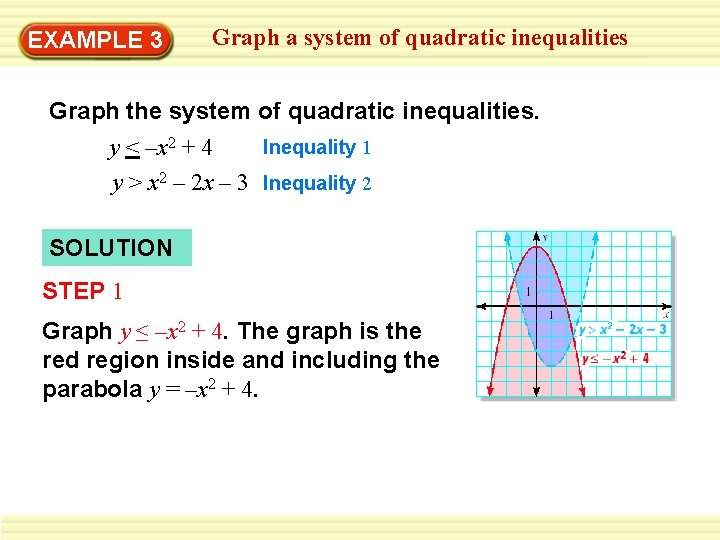EXAMPLE 3 Graph a system of quadratic inequalities Graph the system of quadratic inequalities. Inequality 1 y < –x 2 + 4 y > x 2 – 2 x – 3 Inequality 2 SOLUTION STEP 1 Graph y ≤ –x 2 + 4. The graph is the red region inside and including the parabola y = –x 2 + 4.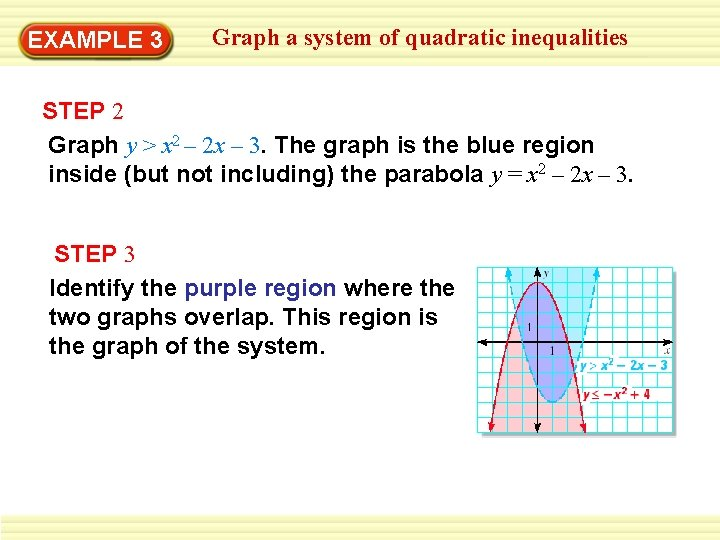EXAMPLE 3 Graph a system of quadratic inequalities STEP 2 Graph y > x 2 – 2 x – 3. The graph is the blue region inside (but not including) the parabola y = x 2 – 2 x – 3. STEP 3 Identify the purple region where the two graphs overlap. This region is the graph of the system.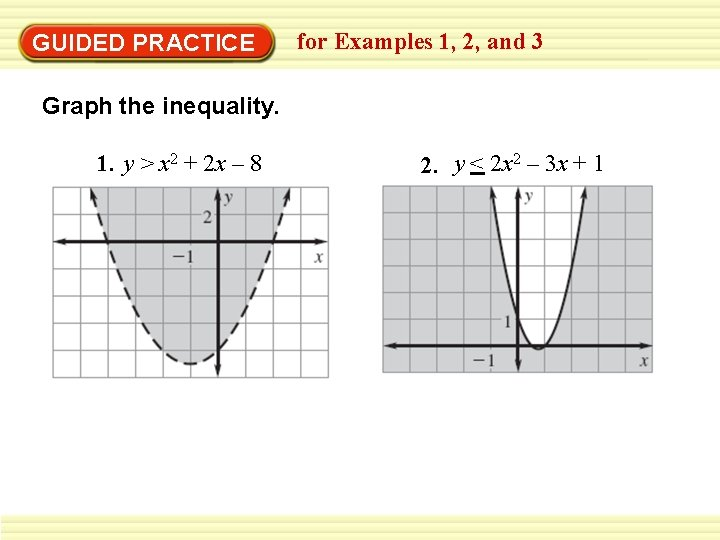GUIDED PRACTICE for Examples 1, 2, and 3 Graph the inequality. 1. y > x 2 + 2 x – 8 2. y < 2 x 2 – 3 x + 1GUIDED PRACTICE for Examples 1, 2, and 3 Graph the inequality. 3. y < –x 2 + 4 x + 2 4. Graph the system of inequalities consisting of y ≥ x 2 and y < –x 2 + 5.